Skip to main content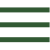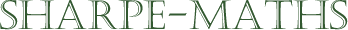Previous topic: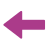Current topic:
Introduction to ratio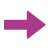Next topic:
Working with ratios

### Ratio

Ratio is one of the best ways of comparing the size of different quantities.

You can compare the sizes of things directly but this turns out not to be very useful as is shown in this example.Here is a photo of me with my grandson Felix. I would estimate that at the time this photo was taken, I was roughly 1m taller than Felix.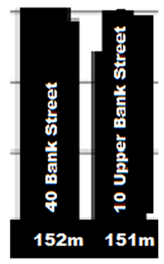Here is a picture of 2 London skyscrapers 40, Bank Street and 10 Upper Bank Street. 40 Bank Street is roughly 1m taller than 10 Upper Bank Street.

Strictly speaking, in absolute terms, the height difference is identical in the 2 situations, but clearly we would generally say that I am a great deal taller than Felix, whereas the two skyscrapers in London are almost exactly the same height.

The reason why this way of comparing size does not work is that it does not take account of the context; the size of the objects.

This is where ratio comes in. It allows us to compare while taking account of the sizes of the objects (or people!) themselves.

There are lots of other uses for ratio which we will look at in the following sections.

### Notation

Ratios are written using the following notation:

$2:1$

The two numbers to be compared are written either side of a colon.

If we return to our previous examples, the ratio of my height to Felix's height was $1.8 : 0.8$, because I am roughly $1.8\text{ m}$ tall and Felix was roughly $0.8\text{ m}$.

If we now look at the skyscrapers, the ratio of the heights of 40 Bank St to 10 Upper Bank St is $152 : 151$, because the two buildings are $151\text{ m}$ and $152\text{ m}$ high respectively.

From this you can see, from a ratio point of view, that I am more than twice Felix's height in this photo, but the two buildings are almost the same height.